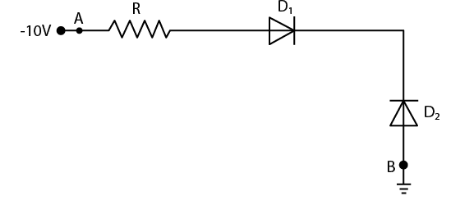# In the figure, assuming the diodes

Question:

In the figure, assuming the diodes to be ideal,(a) D1 is forward biased and D2 is reverse biased and hence current flows from A to B

(b) D2 is forward biased and D1 is reverse biased and hence no current flows from B to A and vice versa

(c) D1 and D2 are both forward biased and hence current flows from A to B

(d) D1 and D2 are both reverse biased and hence no current flows from A to B and vice versa

Solution:

(b) D2 is forward biased and D1 is reverse biased and hence no current flows from B to A and vice versa